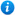# Year 1 Multiplication and Division Bundle

Year 1 Multiplication and Division Bundle

Year 1 maths programme of study - Number - multiplication and division

Pupils are taught to:

• solve one-step problems involving multiplication and division, by calculating the answer using concrete objects, pictorial representations and arrays with the support of the teacher

This year 1 maths bundle introduces pupils to multiplication and division as per the curriculum objectives of the year 1 maths programme of study listed above. It contains 8 PowerPoint presentations with accompanying worksheets. These include:

1. Beginning to Multiply
3. Multiplication Using Arrays
4. Multiplying using a Number Line
5. Beginning to Divide - Grouping
6. Dividing by Grouping
7. Dividing by Sharing
8. Number Line Division
This bundle gives you a saving of 52% when compared to buying each resource individually.

For a full description of each resource please click on the image of the included resources below.

Our Price : £9.99 / 10 Credits## Resources included (8)

£2.50
Beginning to Multiply - Year 1
20 powerpoint slides | 2 worksheets
£1.99
Multiplying Using a Number Line - Year 1
27 powerpoint slides | 3 worksheets
£2.50
Multiplication as Repeated Addition - Year 1
18 powerpoint slides | 2 worksheets
£2.99
Multiplication Using Arrays
53 powerpoint slides | 3 worksheets
£2.99
Beginning to Divide - Grouping
23 powerpoint slides
£1.99
Dividing by Grouping
18 powerpoint slides
£2.99
Dividing by Sharing
24 powerpoint slides
£2.99
Number Line Division
24 powerpoint slides | 2 worksheets

By continuing to use the site, you agree to the use of cookies. You can change this and find out more by following this link